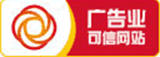## 1.常见的特征工程逻辑

1. 分箱/分桶 离散化

2. log/exp 对数/幂等 math numpy 常见数学运算

3. 特征缩放/归一化/截断

4. 交叉特征生成

5. 分词匹配程度计算

6. 字符串分隔匹配判断 tong

7. 缺省值填充等

8. 数据平滑

9. onehot 编码，hash 编码等

### 二、业务痛点

1. 繁琐，费时费力，极容易出现 python 和 C++ 代码不一致

3. 不一致难以发现，无法测试，无法监控，经常要靠用户投诉反馈，甚至大盘数据异常才能发现

## 1. 业界方案

《推荐系统中模型训练及使用流程的标准化》https://www.infoq.cn
/article/2E6LCqb1GeqFRAjkkjX3

《自主研发、不断总结经验，美团搜索推荐机器学习平台》https://cloud.tencent.com/developer/article/1357309

《京东电商推荐系统实践》https://www.infoq.cn
/article/1OkKmb_gEYNR3YqC9RcW

“模型线上线下一致性问题对于模型效果非常重要，我们使用特征日志来实时记录特征，保证特征的一致性。这样离线处理的时候会把实时的用户反馈，和特征日志做一个结合生成训练样本，然后更新到模型训练平台上，平台更新之后在推送到线上，这样整个排序形成了一个闭环。”

1. 在线特征存储起来给离线用

2. 在线 C++ 代码编译成 so 导出给离线用

3. 根据一份配置生成离线和在线代码

4. 提取公共代码，加强代码复用，等软件工程手段，减少不一致

## (1) .已有方案的缺点

1. 所有在线请求的所有特征，这个存储量数据量很大

2. 算法改代码需要等待后台开发，降低了算法同学的工作效率

3. 特征处理代码的复杂度转移到配置文件中，不一定能充分表达，而且配置格式增加学习成本

4. 就这边真实离线特征处理代码来看，大部分代码都无法抽取出公共代码做复用。

## (2). 翻译器

1. 一条命令 全自动把 Python 翻译成等价 C++

2. 严格等价保证改写，彻底消除不一致

3. 完全去掉重新实现这块工作量，后台开发成本降到 0 ，彻底解放生产力

4. 算法同学继续使用纯 python，开发效率无影响，** 无学习成本 **

5. 并能推广到其他需要 python 改写成后台 C++ 代码的业务场景，解放生产力

## (1). 安装

``pip3 install pythran``

## (2). 写 Python 代码

``import mathimport numpy as npdef zero(n, m):return [*n for col in range(m)]#pythran export matrix_multiply(float list list, float list list)def matrix_multiply(m0, m1):new_matrix = zero(len(m0),len(m1))for i in range(len(m0)):for j in range(len(m1)):for k in range(len(m1)):new_matrix[i][j] += m0[i][k]*m1[k][j]return new_matrix#pythran export arc_distance(float[], float[], float[], float[])def arc_distance(theta_1, phi_1, theta_2, phi_2):"""Calculates the pairwise arc distancebetween all points in vector a and b."""temp = (np.sin((theta_2-theta_1)/2)**2+ np.cos(theta_1)*np.cos(theta_2) * np.sin((phi_2-phi_1)/2)**2)distance_matrix = 2 * np.arctan2(np.sqrt(temp), np.sqrt(1-temp))return distance_matrix#pythran export dprod(int list, int list)def dprod(l0,l1):"""WoW, generator expression, zip and sum."""return sum(x * y for x, y in zip(l0, l1))#pythran export get_age(int )def get_age(age):if age <= 20:age_x = "0_20"elif age <= 25:age_x = "21_25"elif age <= 30:age_x = "26_30"elif age <= 35:age_x = "31_35"elif age <= 40:age_x = "36_40"elif age <= 45:age_x = "41_45"elif age <= 50:age_x = "46_50"else:age_x = "50+"return age_x``

## (3). Python 转成 C++

``pythran -e demo.py -o demo.hpp``

## (4). 写 C++ 代码调用

pythran/pythonic/ 目录下是 python 标准库的 C++ 等价实现，翻译出来的 C++ 代码需要 include 这些头文件

``````#include "demo.hpp"#include "pythonic/numpy/random/rand.hpp"#include <iostream>using std::cout;using std::endl;intmain {pythonic::types::list<pythonic::types::list<double>> m0 = {{2.0, 3.0},{4.0, 5.0}},m1 = {{1.0, 2.0},{3.0, 4.0}};cout << m0 << "*" << m1 << "
=
"<< __pythran_demo::matrix_multiply(m0, m1) << endl<< endl;auto theta_1 = pythonic::numpy::random::rand(3),phi_1 = pythonic::numpy::random::rand(3),theta_2 = pythonic::numpy::random::rand(3),phi_2 = pythonic::numpy::random::rand(3);cout << "arc_distance " << theta_1 << "," << phi_1 << "," << theta_2 << ","<< phi_2 << "
=
"<< __pythran_demo::arc_distance(theta_1, phi_1, theta_2, phi_2) << endl<< endl;pythonic::types::list<int> l0 = {2, 3}, l1 = {4, 5};cout << "dprod " << l0 << "," << l1 << "
=
"<< __pythran_demo::dprod(l0, l1) << endl<< endl;cout << "get_age 30 = " << __pythran_demo::get_age(30) << endl << endl;return 0;}``````

## (5). 编译运行

``g++ -g -std=c++11 main.cpp -fopenmp -march=native -DUSE_XSIMD -I /usr/local/lib/python3.6/site-packages/pythran/ -o pythran_demo./pythran_demo``

## (1). 介绍

pythran 的 manual 挺详细：

## (2). 功能

pythran 并不支持完整的 python， 只支持 python 语言特性的一个子集:

• polymorphic functions 多态函数(翻译成 C++ 的泛型模板函数)

• lambda

• list comprehension 列表推导式

• map, reduce 等函数

• dictionary, set, list 等数据结构

• exceptions 异常

• file handling 文件处理

• 部分 numpy

• classes 类

• polymorphic variables 可变类型变量

## (3). 支持的数据类型和函数

pythran export 可以导出函数和全局变量。支持导出的数据类型，BNF 定义是：

`` argument_type = basic_type| (argument_type+) # this is a tuple| argument_type list # this is a list| argument_type set # this is a set| argument_type + # this is a ndarray, C-style| argument_type [::]+ # this is a strided ndarray| argument_type [:,...,:]+ # this is a ndarray, Cython style| argument_type [:,...,3]+ # this is a ndarray, some dimension fixed| argument_type:argument_type dict # this is a dictionarybasic_type = bool | byte | int | float | str | None | slice| uint8 | uint16 | uint32 | uint64 | uintp| int8 | int16 | int32 | int64 | intp| float32 | float64 | float128| complex64 | complex128 | complex256``

pythran/pythonic/include/types/ 下面，可以看到比如 dict 实际就是封装了一下 std::unordered_map

### 五、pythran 的基本原理

1. python 代码解析成抽象语法树 AST 。用 python 标准库自带的的 ast 模块实现

2. 代码优化。在 AST 上做优化，有多种 transformation pass，比如 deadcode_elimination 死代码消除，loop_full_unrolling 循环展开 等。还有 Function/Module/Node 级别的 Analysis，用来遍历 AST 供 transformation 利用。

3. 后端，实现代码生成。目前有 2 个后端，Cxx / Python， Cxx 后端可以把 AST 转成 C++ 代码（ Python 后端用来调试）。

1. 字符串处理能力欠缺，缺少 str.encode/str.decode 对 utf8 的支持

2. 缺少正则表达式 regex 支持

### 六、相关文章

《京东电商推荐系统实践》https://www.infoq.cn
/article/1OkKmb_gEYNR3YqC9RcW

《自主研发、不断总结经验，美团搜索推荐机器学习平台》https://cloud.tencent.com/developer/article/1357309

《推荐系统中模型训练及使用流程的标准化》https://www.infoq.cn
/article/2E6LCqb1GeqFRAjkkjX3

numbahttp://numba.pydata.org

7

8

c语言中文官网-零基础c++从入门到精通pdf、c语言编译器、C/C++开发工具、c语言入门自学零基础、c++入门自学、c/c++软件下载、c语言编程软件、c语言自学免费网站、c语言零基础自学视频教程、c++手机编程软件、c语言入门程序设计、初级编程视频教程、c语言在线编程平台、C语言线上作业网站。